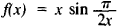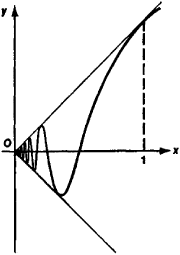# length

(redirected from stride length)
Also found in: Dictionary, Thesaurus, Medical.

## length

1. Prosody Phonetics the metrical quantity or temporal duration of a vowel or syllable
2. the distance from one end of a rectangular swimming bath to the other
3. Prosody the quality of a vowel, whether stressed or unstressed, that distinguishes it from another vowel of similar articulatory characteristics. Thus in English beat is of greater length than in English bit
4. Cricket the distance from the batsman at which the ball pitches
5. Bridge a holding of four or more cards in a suit

## Length

A one-dimensional extension in space. Length is one of the three fundamental physical quantities, the others being mass and time. It can be measured by comparison with an arbitrary standard; the specific one in most common usage is the international meter. In 1983, at the meeting of the Conférence Général des Poids et Mésures, the meter was redefined in terms of time and the speed of light: “The meter is the length of the path traveled by light in vacuum during a time interval of 1/299 792 458 of a second.” This definition defines the speed of light to be exactly 299 792 458 m/s, and defines the meter in terms of the most accurately known quantity, the second. See Light, Mass, Time

## Length

a numerical characteristic of the extent of curves. The concept of length is defined differently for different cases. (1) The length of a line segment is the distance between its end points, measured by some segment taken as a unit of length. (2) The length of a polygonal line is the sum of the lengths of its components. (3) The length of a simple arc is the limit of the lengths of polygonal lines inscribed in the arc, when the number of components increases indefinitely and the maximum length of the components tends to zero. (4) The length of a continuous curve consisting of a finite number of simple arcs is equal to the sum of the lengths of these arcs. For example, the circumference of a circle can be obtained as the limit of the perimeters of inscribed regular polygons when the number of their sides is doubled indefinitely; it is equal to 2πR where R is the radius of the circle. Any continuous curve has finite or infinite length. If its length is finite, then the curve is said to be rectifiable. The graph (see Figure 1) of the functionfor 0 <x ≤ 1 and f(x) = 0 when x = 0 is an example of a nonrectifiable curve; here the lengths of the inscribed polygonal lines increase beyond all bound as the length of the components tend to zero. If the equation of a plane curve has the form y =f(x) (a ^ x ^ b)m rectangular coordinates and the function f(x) has a continuous derivative f’(x), then the length of the curve is given by the integralThe length of a curve given in parametric form and the length of a space curve can be expressed in a similar manner.Figure 1

To calculate the length of a curve the mathematicians of antiquity essentially used lengths of polygonal lines and passage to the limit. For them, however, such passage to the limit was only a method for calculating the length of a curve and not for defining the concept of the length of a curve, since they apparently perceived the latter as one of the elementary mathematical concepts. The necessity of defining the length of a curve became clear only in the first half of the 19th century. A full elucidation of the problem was achieved by C. Jordan. In differential geometry the length of a curve is also defined on a surface or in an arbitrary Riemannian space.

### REFERENCES

Lebesgue, H. Ob izmerenii velichin, 2nd ed. Moscow, 1960. (Translated from French.)
Fikhtengol’ts, G. M. Kurs differentsial’nogo i integral’nogo ischisleniya, 7th ed. vol. 2. Moscow, 1969.

## length

[leŋkth]
(mechanics)
Extension in space.
References in periodicals archive ?
Assessment of stride length (A), step length (B), stride width (C), and sciatic functional index (D) of Wistar rats of different ages.
Students will measure and record the stride length, by measuring the distance from left foot to left foot, or right foot to right foot and record.
On examination, walking speed, stride length, and heel strike significantly increased, with resolution of FoG.
Velocity, stride length, single leg support time and swing time
The present findings suggest stride height and stride length are not associated with displacement of the head during the baseball swing in experienced collegiate baseball players.
In contrast, Kalron (15) found that modifications in spatiotemporal parameters of gait (eg, step and stride length) were not closely related to symptomatic fatigue in individuals with MS.
Repeated measures ANOVA was used for within-group comparison of obese group participants' walking speed, cadence, step length, step width, and stride length across baseline, week 4, week 8, and week 12 of the study while paired /-test with Bonferroni adjustment was used for post hoc analysis.
In order to improve the accuracy of step detection and stride length estimation, the IMU is usually placed on the foot or leg.
Biomechanical analysis revealed that compared to conventional heel-toe running, Pose running resulted in shorter stride lengths and smaller vertical oscillations of the pelvis.
Stride length was estimated using the distance measured between first and second initial contact of the left foot (5th metatarsal).
The addition of a DTc (subtracting 7) while walking caused changes of gait parameters in both stroke (men and women) patient groups: a slight reduction of walking speed, cadence and stride length with an increase of gait cycle parameters (stride time, double support percentage) and gait variability parameters could be detected at both women and men.
When compared with control subjects, patients with PAD had significantly decreased walking velocity (p < 0.001), cadence (p < 0.001), stride length (p < 0.001), and stance phase percent of the total gait cycle (p = 0.02).

Site: Follow: Share:
Open / Close